# Torque of a spinning flywheel

Hello, I would like to know the amount of torque required when the flywheel starts? I know that once at speed the flywheel doesn't require torque. I would also like to know what size of slip ring induction motor to run as the below mentioned speed.

The weight of the flywheel is= 6500 kg
( wt. shaft, flywheel boss and wall 2700 kg + mass 3800 kg = total 6500 kg )
RPM= 1550
Diameter=2200mm

russ_watters
Mentor
The amount of torque required depends on its moment of inertia and how fast you want it to spin up.

jack action
Gold Member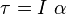http://en.wikipedia.org/wiki/Angular_acceleration#Equations_of_motion

Last edited by a moderator:
...and angular acceleration alpha is approximately closely enough by delta angular velocity / delta time.

The amount of torque required depends on its moment of inertia and how fast you want it to spin up.
Thanks russ_watters, isn't how fast I want the fly wheel to spin the RPM? And if you don't mind how am I supposed to calculate the moment of inertia? Sorry for the trouble as am still an amateur at this thanks

Thanks russ_watters, isn't how fast I want the fly wheel to spin the RPM? And if you don't mind how am I supposed to calculate the moment of inertia? Sorry for the trouble as am still an amateur at this thanks
By RPM we usually mean the speed of rotation after the flywheel is up to speed. russ_watters and the other posters are talking about acceleration. How long do you want it to take to go from 0 RPM to max RPM? The shorter the time, the more torque you'll need.

You can find the moment of inertia on this table: http://en.wikipedia.org/wiki/List_of_moments_of_inertia
Note that multiple values are listed for each shape. The subscript tells you which axis you are rotating about.

jack action
Gold Member
Thanks russ_watters, isn't how fast I want the fly wheel to spin the RPM?

No, it's the angular accelration α that I mentioned in my post. As tygerdawg mentioned, the average angular acceleration is αavg = ( RPMfinal - RPMinitial ) / time.

And if you don't mind how am I supposed to calculate the moment of inertia? Sorry for the trouble as am still an amateur at this thanks

This the I in the equation of my previous post.

If it is a solid disc: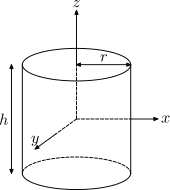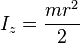If it is a ring: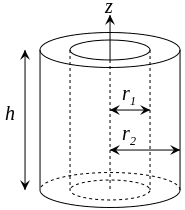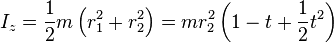For other basic shapes: http://en.wikipedia.org/wiki/List_of_moments_of_inertia

Calculation method for more complex shapes: http://en.wikipedia.org/wiki/Moment_of_inertia#Calculating_moment_of_inertia_about_an_axis

•jackwhirl